Share

# Let L Be a Line and P Be a Point Not on L. Through P, Draw a Line M Parallel to L. Now Join P to Any Point Q on L. Choose Any Other Point R on M. Through R, Draw a Line Parallel to Pq. Let this Meet L at S. What Shape Do the Two Sets of Parallel Lines Enclose? - CBSE Class 7 - Mathematics

ConceptConstruction of a Line Parallel to a Given Line, Through a Point Not on the Line

#### Question

Let l be a line and P be a point not on l. Through P, draw a line m parallel to l. Now join P to any point Q on l. Choose any other point R on m. Through R, draw a line parallel to PQ. Let this meet l at S. What shape do the two sets of parallel lines enclose?

#### Solution

The steps of construction are as follows.

1) Draw a line l and take a point A on it. Take a point P not on l and join A to P.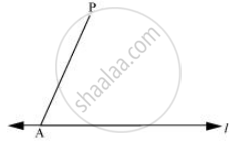2) Taking A as centre and with a convenient radius, draw an arc cutting l at B and AP at C.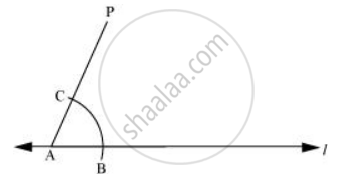3) Taking P as centre and with the same radius as before, draw an arc DE to intersect AP at F.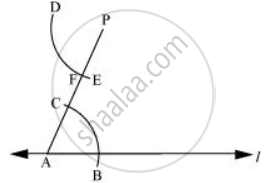4) Adjust the compasses up to the length of BC. Without changing the opening of compasses and taking F as the centre, draw an arc to intersect the previously drawn arc DE at point G.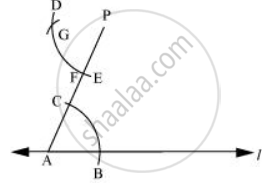5) Join P to G to draw a line m. Line m will be parallel to line l.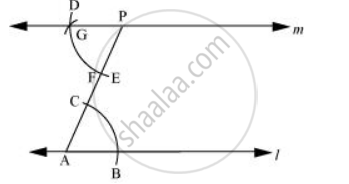6) Join P to any point Q on line l. Choose another point R on line m. Similarly, a line can be drawn through point R and parallel to PQ.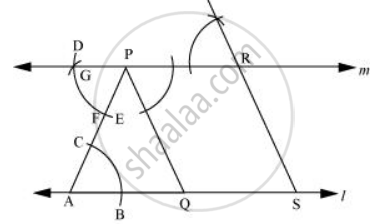Let it meet line l at point S.

In quadrilateral PQSR, opposite lines are parallel to each other.

PQ || RS and PR || QS

Thus, the quadrilateral PQSR is a parallelogram.

Is there an error in this question or solution?

#### APPEARS IN

NCERT Solution for Mathematics for Class 7 (2018 to Current)
Chapter 10: Practical Geometry
Ex. 10.10 | Q: 3 | Page no. 196
Solution Let L Be a Line and P Be a Point Not on L. Through P, Draw a Line M Parallel to L. Now Join P to Any Point Q on L. Choose Any Other Point R on M. Through R, Draw a Line Parallel to Pq. Let this Meet L at S. What Shape Do the Two Sets of Parallel Lines Enclose? Concept: Construction of a Line Parallel to a Given Line, Through a Point Not on the Line.
S